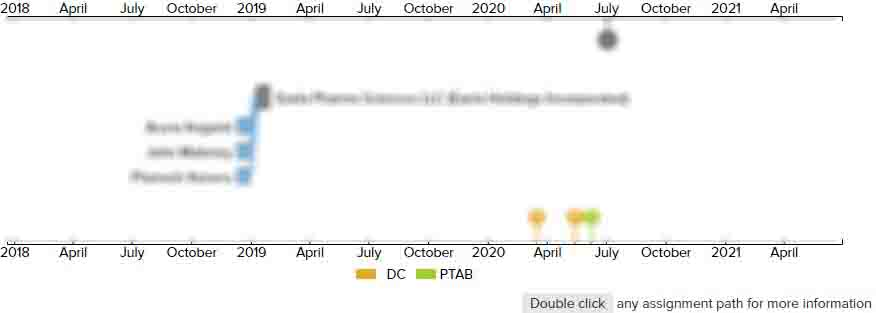# Intermediates useful for the preparation of antihistaminic piperidine derivatives

• US 6,555,689 B2
• Filed: 12/01/2000
• Issued: 04/29/2003
• Est. Priority Date: 06/25/1993
• Status: Expired due to Fees
##### First Claim
Patent Images

1. A process for preparing a compound of the formula whereinW represents —

• C(═

O)—

or —

CH(OH)—

;

R1 represents hydrogen or hydroxy;

R2 represents hydrogen;

or R1 and R2 taken together form a second bond between the carbon atoms bearing R1 and R2;

n is an integer of from 1 to 5;

m is an integer 0 or 1;

R3 is —

COOH or —

COOalkyl wherein the alkyl moiety has from 1 to 6 carbon atoms and is straight or branched;

each of A is hydrogen or hydroxy; and

pharmaceutically acceptable salts and individual optical isomers thereof, with the proviso that where R1 and R2 are taken together to form a second bond between the carbon atoms bearing R1 and R2 or where R1 represented hydroxy, m is an integer 0, comprising the steps of;

(a) reacting a cumene compound of the formula wherein A is as defined above with a ω

-halo compound of the formula wherein B is halo or hydroxy, Hal represents Cl, Br or I and n is as defined above, in the presence of a suitable Lewis acid to produce a ω

-halo cumylketone compound;

(b) reacting the ω

-halo cumylketone compound with a suitable halogenating agent to give a ω

-halo-halocumylketone compound;

(c) reacting the ω

-halo-halocumylketone compound compound with a suitable cyanating agent to give a ω

-halo-cyanocumylketone compound;

(d) reacting the ω

-halo-cyanocumylketone compound with an appropriate straight or branched C1-C6 alcohol in the presence of a suitable anhydrous acid to give a ω

-halo-α

-keto-α

-dimethylphenylacetic acid imidate compound;

(e) reacting the ω

-halo-α

-keto-α

-dimethylphenylacetic acid imidate compound with water to give a ω

-halo-α

-keto-α

-dimethylphenylacetic acid ester compound;

(f) reacting the ω

-halo-α

-keto-α

-dimethylphenylacetic acid ester compound with a piperidine compound of the formula

wherein R1, R2 and m are as defined above in the presence of a suitable non-nucleophilic base to produce a ω

-piperidine-α

-keto-α

-dimethylphenyl derivative of formula (I) wherein R3 is COOalkyl and W is —

C(═

O)—

;

(g) optionally hydrolyzing the ω

-piperidine- α

-keto-α

-dimethylphenyl derivative of formula (I) wherein R3 is COOalkyl and W is —

C(═

O)—

to produce a ω

-piperidine- α

-hydroxy-α

-dimethylphenyl derivative of formula (I) wherein R3 is COOH and W is —

C(═

O)—

;

(h) optionally reacting the ω

-piperidine-α

-keto-α

-dimethylphenyl derivative of formula (I) wherein R3 is COOalkyl and W is —

C(═

O)—

or the ω

-piperidine-α

-keto-α

-dimethylphenyl derivative of formula (I) wherein R3 is COOH and W is —

C(═

O)—

with a suitable reducing agent to produce a ω

-piperidine-α

-hydroxy-α

-dimethylphenyl derivative of formula (I) wherein R3 is —

COOH and W is —

CH(OH)—

or the ω

-piperidine-α

-hydroxy-α

-dimethylphenyl derivative of formula (I) wherein R3 is —

COOalkyl and W is —

CH(OH)—

; and

(i) optionally reacting the ω

-piperidine-α

-hydroxy-α

-dimethylphenyl derivative of formula (I) wherein R3 is —

COOH and W is —

CH(OH)—

or the appropriate ω

-piperidine-α

-keto-α

-dimethylphenyl derivative of formula (I) wherein R3 is —

COOH and W is —

C(═

O)—

with an appropriate straight or branched C1-C6 alcohol in the presence of a suitable acid to produce a ω

-piperidine-α

-hydroxy-α

-dimethylphenyl derivative of formula (I) wherein R3 is —

COOalkyl and W is —

CH(OH)—

or a ω

-piperidine-α

-keto-α

-dimethylphenyl derivative wherein R3 is —

COOalkyl and W is —

C(═

O)—

; and

(j) optionally reacting the ω

-piperidine-α

-keto-α

-dimethylphenyl derivative of formula (I) wherein R3 is —

COOH and W is —

C(═

O)—

, the ω

-piperidine-α

-keto-α

-dimethylphenyl derivative of formula (I) wherein R3 is —

COOalkyl and W is —

C(═

O)—

, the ω

-piperidine-α

-hydroxy-α

-dimethylphenyl derivative of formula (I) wherein R3 is —

COOH and W is —

CH(OH)—

or the ω

-piperidine-α

-hydroxy-α

-dimethylphenyl derivative of formula (I) wherein R3 is —

COOalkyl and W is —

CH(OH)—

with an appropriate deprotecting reagent, with the proviso that each of the hydroxy groups present in the compounds described in steps a-i are optionally protected or unprotected.

• ##### 13 Assignments
Timeline View
Assignment View×
×